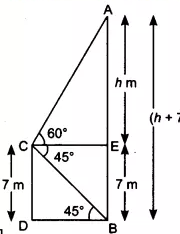Q

# From the top of a 7 m high building, the angle of elevation of the top of a cable tower is 60° and the angle of depression of its foot is 45°. Determine the height of the tower.

12. From the top of a 7 m high building, the angle of elevation of the top of a cable tower is 60° and the angle of depression of its foot is 45°. Determine the height of the tower.

ViewsLet the height of the cable tower be (AB = )m
Given,
Height of the building is 7 m and angle of elevation of the top of the tower  , angle of depression of its foot .

According to question,

In triangle ,

since DB = CE = 7 m

In triangle ,

Thus, the total height of the tower equal to

Exams
Articles
Questions